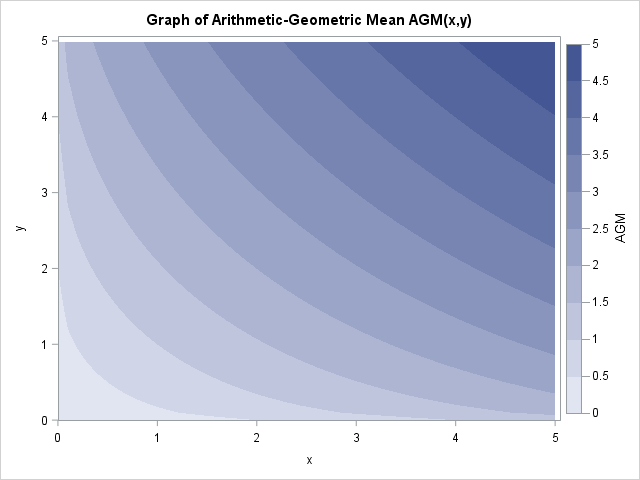All statisticians are familiar with the classical arithmetic mean. Some statisticians are also familiar with the geometric mean. Whereas the arithmetic mean of n numbers is the sum divided by n, the geometric mean of n nonnegative numbers is the n_th root of the product of the numbers. The geometric mean is always less than the arithmetic mean.

Did you know that there is a hybrid quantity, called the arithmetic-geometric mean, which is defined by combining the two quantities? The arithmetic-geometric mean is only defined for two positive numbers, x and y. It is defined as the limit of an alternating iterative process:

• Define a1 = (x + y)/2 and g1 = sqrt(x y) to be the arithmetic and geometric means, respectively.
• Iteratively define an+1 = (an + gn)/2 and gn+1 = sqrt(an gn).

Interestingly, this process always converges. The number that it converges to is called the arithmetic-geometric mean of x and y, which is denoted by AGM(x,y). The AGM is between the geometric mean and the arithmetic mean. I am not aware of a multivariate generalization of the AGM function.

In SAS you can use the SAS/IML language or PROC FCMP to create a user-defined function that computes the arithmetic-geometric mean. Since the AGM is not a vector operation, I show the PROC FCMP approach:

```proc fcmp outlib=work.funcs.MathFuncs; function AGM(x, y); if x<0 | y<0 then return( . ); epsilon = 1e-10; /* precision of computation */ a = mean(x, y); g = geomean(x,y); do while (abs(a - g) > epsilon); a_new = mean(a, g); g_new = geomean(a, g); a = a_new; g = g_new; end; return( mean(a, g) ); endsub; quit;   /* test the function */ options cmplib=work.funcs; /* define location of function */ data _NULL_; agm = AGM(1, 0.8); put agm 18.14; run;```
` 0.89721143211504`

An example of calling the new AGM function is shown for the two numbers 1 and 0.8. The arithmetic mean of 1 and 0.8 is 0.9. The geometric mean of 1 and 0.8 is sqrt(0.8) = 0.8944. The computation shows that the arithmetic-geometric mean is 0.8972, which is between the arithmetic and geometric means.

The following program computes the graph of the AGM function and displays a contour plot of the result. Note that AGM(x, x) = x for any nonnegative value of x.

```data grid; do x = 0 to 5 by 0.1; do y = 0 to 5 by 0.1; agm = AGM(x, y); output; end; end; run;   /* see http://blogs.sas.com/content/iml/2012/07/02/create-a-contour-plot-in-sas.html */ proc sgrender data=grid template=ContourPlotParm; dynamic _TITLE="Graph of Arithmetic-Geometric Mean AGM(x,y)" _X="x" _Y="y" _Z="AGM"; run;```So, what is the arithmetic-geometric mean good for? The AGM seems mainly useful in applied mathematics and number theory for computing elliptic functions and elliptic integrals. I am not aware of any applications to statistics, perhaps because the AGM is not defined for a sample that contains n elements when n > 2.

Although the AGM function is not used in statistics, the iterative algorithm that defines the AGM is a technique that I have seen often in computational statistics. When a researcher wants two conditions to hold simultaneously, one possible method is to alternately solve for each condition and prove that this iterative process converges to a solution that satisfies both conditions simultaneously. The iterative process can be an effective way to implement an optimization problem if each sub-optimization is simple.

In multivariate statistics, this technique is used in the method of alternating least squares, which is used in several SAS procedures such as PROC TRANSREG, VARCLUS, and MDS. In linear algebra, this technique is used in the method of alternating projections. I have used this technique to compute the closest correlation matrix to an arbitrary matrix.

One last interesting fact. There is another kind of mean called the harmonic mean. You can compute the arithmetic-harmonic mean by applying the same iterative algorithm, but replace the GEOMEAN function with the HARMEAN function. The process converges and the arithmetic-harmonic mean converges is equal to the geometric mean! Thus although this two-step definition might seem contrived, it is "natural" in the sense that it produces the geometric mean (of two numbers) from the arithmetic and harmonic means.

The post The arithmetic-geometric mean appeared first on The DO Loop.